# CLAST Math On-Line Practice Quiz

### (Chapter 2 - Form A: Algebra Skills)

```CLAST Algebra Skill Objectives

Add, subtract, multiply and divide real numbers
Apply the order-of-operations agreement
Use scientific notation
Solve linear equations and inequalities
Use formulas to compute results
Find particular values of a function
Find the roots of a quadratic equation
Solve a system of two linear equations in two unknowns
Use properties of operations correctly
Determine whether a number is among the solutions of a given equation or inequality
Recognize statements and conditions of proportionality and variation
Identify regions of the coordinate plane that correspond to specific conditions and vice versa
Use applicable properties to select equivalent equations or inequalities
Solve real-world problems involving the use of variables
Solve problems that involve the structure and logic of algebra

```

Enter First Name followed by a space then your Last Name here

## Question 1

Simplify:  7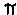+ 5- 5

a) 122 - 5
b) 7c) 12- 5
d) 2+ 7

## Question 2

Add: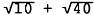=

a)b)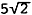c) 1700
d) 20

## Question 3

Substract: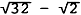=

a)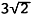b) 28
c) 4
d)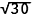## Question 4

Multiply: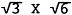=

a) 18
b) 3
c) 9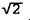d) 3## Question 5

Divide: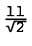=
a)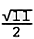b)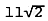c)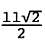d)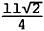## Question 6

Find:  (6.0 x 1020 ) (4.2 x 10-12)

a) 25,200,000,000
b) -2,520,000,000
c) 252,000,000
d) 2,520,000,000

## Question 7

Find:  0.000486 ÷ 243,000

a) 2.00 x 101
b) 2.00 x 109
c) 2.00 x 10-1
d) 2.00 x 10-9

## Question 8

Find:  (28.2 x 102) ÷ (9.4 x 102 )

a) -3.0
b) 0.30
c) 3.0
d) 30

## Question 9

Find:  1/2 - 2 (1/2 + 3)

a) 2½
b) 6½
c) 3/4
d) -6½

## Question 10

Find:  10t - 5t x 4 + 18t2 ÷ 6 x 5

a) 15t2+ 20t
b) 15t2 - 10t
c) 3/5 t2 + 20t
d) 3/5 t2 - 10t

## Question 11

Choose the expression equivalent to the following:
9(8) + 9(4)

a) (9 + 9) (8 + 4)
b) 9 (8 + 4)
c) 18 (8) (4)
d) 9 (9) + 8 (4)

## Question 12

Choose the expression equivalent to the following:
4 + 14 + 16

a) 4 (14 + 16)
b) 16 - 14 - 4
c) 4 (14) + 16
d) 14 + 4 + 16

## Question 13

Choose the statement that is not true for all real numbers.

a) 3xy (5x + y) = 3xy (5y + x)
b) (5a)b = 5(ab)
c) ( x + y ) ( x - y) = ( x - y) ( x + y)
d) 3 (a) + 3 (b) = 3( a + b)

## Question 14

Choose the inequality equivalent to -8  <  - 8x - 24 < 8 .

a) -4 < <  -2
b) x > 4
c) -2 <<  4
d) x  <  -2

## Question 15

Solve for a:    3(a + 10) = 8 [a - (7 - a)]

a) -6 8/13
b) 6 8/13
c) -34
d) 2 4/13

## Question 16

Solve for a:   7a = 19(a - [14a + (18a - 1) ] ) + 17

a) a = 1/298
b) a = - 9/149
c) a = - 1/298
d) a = 9/149

## Question 17

Solve for b:   b - 7 < 2b + 20

a) b > -27
b) b = -27
c) b > 27
d) b < -27

## Question 18

Solve for x:    4x - 5x + 18 > 4x - (14 - 7x)

a) x < - 16/5
b) x < 8/3
c) x > - 16/5
d) x > 8/3

## Question 19

For each of the statements below, determine whether -1 is a solution.
i.   | x - 1 | = 0
ii.  (t - 3) ( t - 6 ) < 6
iii. y2+ 4y + 16 = 13

a) ii and iii only
b) ii only
c) iii only
d) i only

## Question 20

For each of the statements below, determine whether 1/2 is a solution.
i.   2x - 1 < 0
ii.  (4y - 1) (y - 4) = 0
iii. 2t - 2 = -2t

a) i and iii only
b) All of the statements
c) ii and iii only
d) i only

## Question 21

For each of the statements below, determine whether 3 is a solution:
i.   x3 > 0
ii.  (x - 3) (x + 4) = 0
iii. 3(x - 8) > 0, given x > 8

a) i only
b) All of the statements
c) i and ii only
d) ii and iii only

## Question 22

If a = (b + 2)2and b = 8, find a .

a) 68
b) 64
c) 10
d) 100

## Question 23

The formula for converting a Fahrenheit temperature to Celsius is C = 5/9 (F - 32º). What is the temperature on the Celsius scale when the Fahrenheit temperature is 59º ?

a) 51º
b) 15º
c) 135º
d) 1º

## Question 24

The formula for finding the simple interest (I) on a loan is I = PRT. How much interest will Bill pay on his car loan if he finances \$3,000 (P) at a 13% simple interest rate (R) for 5 years (T) ?

a) \$78
b) \$1950
c) \$195
d) \$390

## Question 25

Find f (2) given f (x) = 3x2 - x + 17

a) 21
b) 25
c) 27
d) 10

## Question 26

Find f (-1) given f (x) = 3x3 - x2 - 22

a) -27
b) -24
c) -26
d) 26

## Question 27

Which is a linear factor of 3x2 + 7x + 4 ?

a) x + 2
b) x - 2
c) 3x + 4
d) 3x - 5

## Question 28

Which is a linear factor of 2x2 - 4x - 6 ?

a) x + 2
b) x + 1
c) x + 3
d) x - 2

## Question 29

Which is a linear factor of 2x2 - 512 ?

a)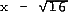b)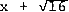c) x - 16
d) x - 256

## Question 30

Use the quadratic formula to solve:   2x2+ 7x + 1 = 0

a)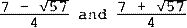b)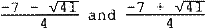c)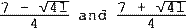d)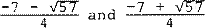## Question 31

Find the real roots of the equation:   3x2- 1 = 5x

a)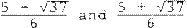b)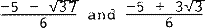c)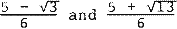d)## Question 32

Find the real roots of the equation:   4x2 = 7x + 1

a)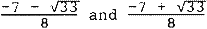b)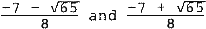c)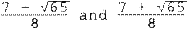d)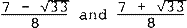## Question 33

Solve the system:
3x - 2y = 1
4x - y = -2

a) { ( x, y) | y = 3/2 x - 1/2}
b) (-1, -2 )
c) ( -1, 2 )
d) The empty set

## Question 34

Solve the system:
2x + y = -7
-2x - y = -5

a) (-3 , -1)
b) { ( x, y) | y = -2x - 7}
c) The empty set
d) (3 , -1)

## Question 35

Solve the system:
4y + x = 3
x + 4y = 3

a) { ( x, y) | y = - 1/4 x + 3/4 }
b) (-1, -1)
c) The empty set
d) ( -1 , 1)

## Question 36

Which shaded region identifies the portion of the plane in which x > -1 and y < 0 ?

a)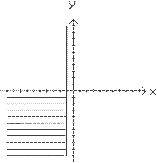b)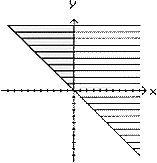c)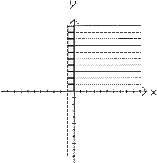d)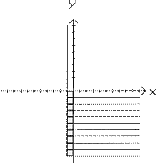## Question 37

What conditions correspond to the shaded region of the plane shown below ?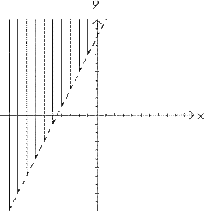a) x < 9, y > 9, and y > x
b) y > 2x + 9
c) x < 9 > 9
d) y = -x

## Question 38

Which option gives the condition(s) that corresponds to the shaded region of the plane shown below?a) y = x
b) x > -3 , y < -3 and y < x
c) x > -3 and y < -3
d) y > x - 3

## Question 39

Two machines can complete 5 tasks every 4 days. Let t represent the number of tasks these machines can complete in a 30-day month. Select the correct statement of the given condition.

a) t/5 = 2/15
b) t/4 = 3
c) 5/4 = t/30
d) 4/5 = t/30

## Question 40

There are 3 male students to every 9 female students at a local school. Select the statement of the condition when there are 33 male students.

a) 3/9 = X/33
b) 3/9 = 33/X
c) 33/3 = 9/X
d) 9/3 = 33/X

## Question 41

Nine pounds of grass seed will cover 120 square feet. Let p represent the number of pounds of seed needed to cover 580 square feet. Select the correct statement of the given condition.

a) p/9 = 120/580
b) 9/120 = 580/p
c) 9/120 = p/580
d) 120/9 = p/580

## Question 42

The area of a set of rectangular pictures is held constant while the length and width may change. If the length is 12 when the width is 2, select the statement of the condition when the width is 5 .

a) L/12 = 2/5
b) L/12 = 5/2
c) 12/2 = L/5
d) L/5 = 2/12

## Question 43

The cost of a long-distance phone cell from New York to Paris is defined by C(t) = 0.80( t -1)+ 1.50, where the cost is \$1.50 for the first minute and \$ 0.85 for each additional minute. Find the cost of a 6-minute phone call.

a) \$ 5.75
b) \$ 4.25
c) \$ 6.60
d) \$ 5.10

## Question 44

The horsepower that a rotating shaft can safely transmit varies jointly as the cube of its diameter d and the number n of revolutions it makes per minute. If a 2-inch shaft at a speed of 2000 revolutions per minute can safely transmit 200 horsepower, find the horsepower that a 3-inch shaft can safely transmit at a speed of 2300 revolutions per minute.

a) 1553 hp
b) 776 hp
c) 259 hp
d) 518 hp

## Question 45

The difference between a number and two more than four times the number is eleven . What equation could be used to find x, the number?

a) x - (2x + 4) = 11
b) x - (4x + 2) = 11
c) x - 4x + 2 = 11
d) x + (4x - 2) = 11

## Question 46

The sum of a number and 8 is 32 . What equation could be used to find x, the number?

a) x + 32 = 8
b) 8 - x = 32
c) x + 8 = 32
d) x - 8 = 32

When you have completed this quiz, press the ALL DONE-CHECK TEST button below to check the test! Then you may go back and change the incorrect answers before you submit the test. You may check the answers as many times as you need until you achieve a perfect grade. Sometimes the pop-up box does not appear when you click on the button after checking the test the first time. If this happens, minimize the test and the answer box will be behind the browser. close the box and maximize the test to continue checking the test and finally submitting it.

When you have finished checking the test, press the SUBMIT button to send answers to your instructor